# 17. 机器学习 - 随机森林

[TOC]Hi，你好。我是茶桁。

## 随机森林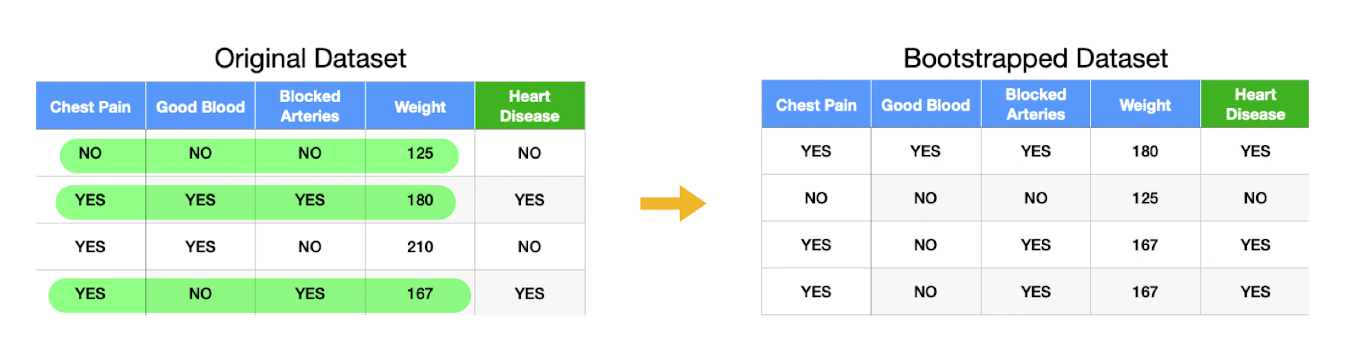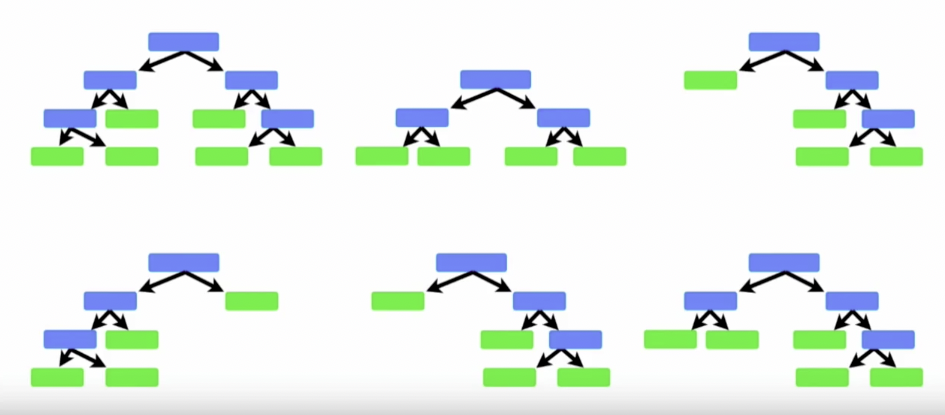1. 使用随机森林来预测。
2. 在预测之前呢，我们使用 Out-Of-Bagging 样本来评估我们的模型。这个 bagging 就是袋子，就是我们从袋子里随机取东西去衡量。
3. 使用评估结果，我们可以选择合适的变量数。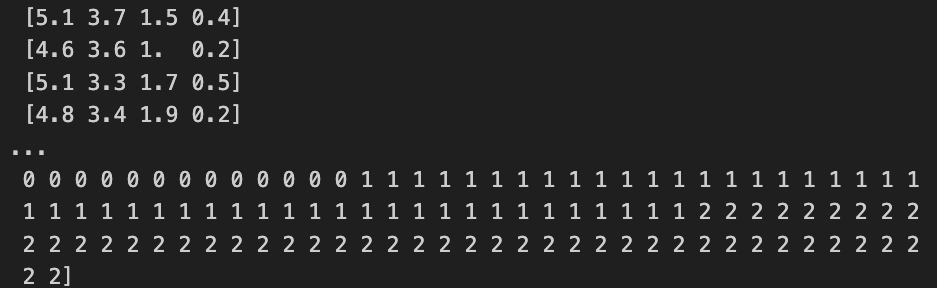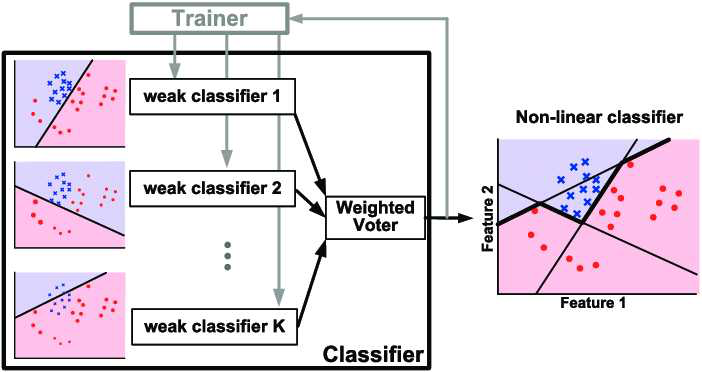Adaboost 就是在随机森林的权重思路上做了一个优化，它的示意图也是有多个 weak classifier, 然后最后有一个 Weighted Voter, 这是一个权重的投票，这个就和我们上面加权的那部分代码非常的类似。只不过它在这里做了个细化：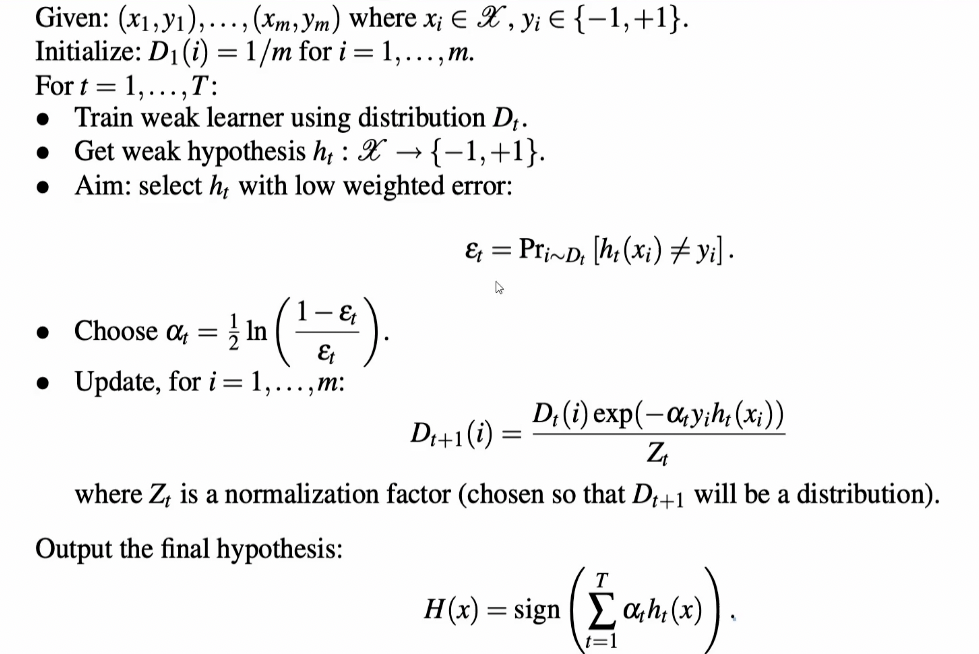$H(x) = sign(\sum_{t=1}^T\alpha_th_t(x))$

\begin{align*} \alpha_t = \frac{1}{2}ln(\frac{1-\varepsilon_t}{\varepsilon_t}) \end{align*}

\begin{align*} \varepsilon_t = Pr_{i\sim D_t}[h_t(x_i)\ne y_i] \end{align*}

$D_{t+1}(i) = \frac{D_t(i)exp(-\alpha_ty_ih_t(x_i))}{Z_t}$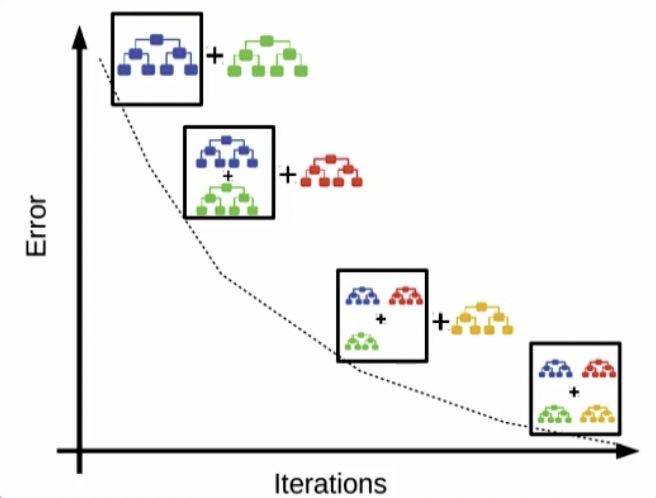$loss(p, q) = -\sum_{i\in output classes}p(x)logq(x)$

$f(x) = f(x) + learning\_rate \cdot h(x) \\$

$Boosted Ensemble = First Tree + \eta \cdot Second Tree \\ loss(Boosted Ensemble) < loss(First Tree)$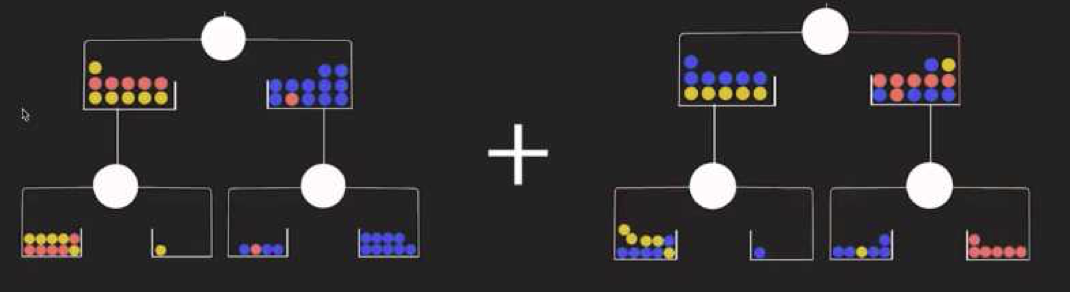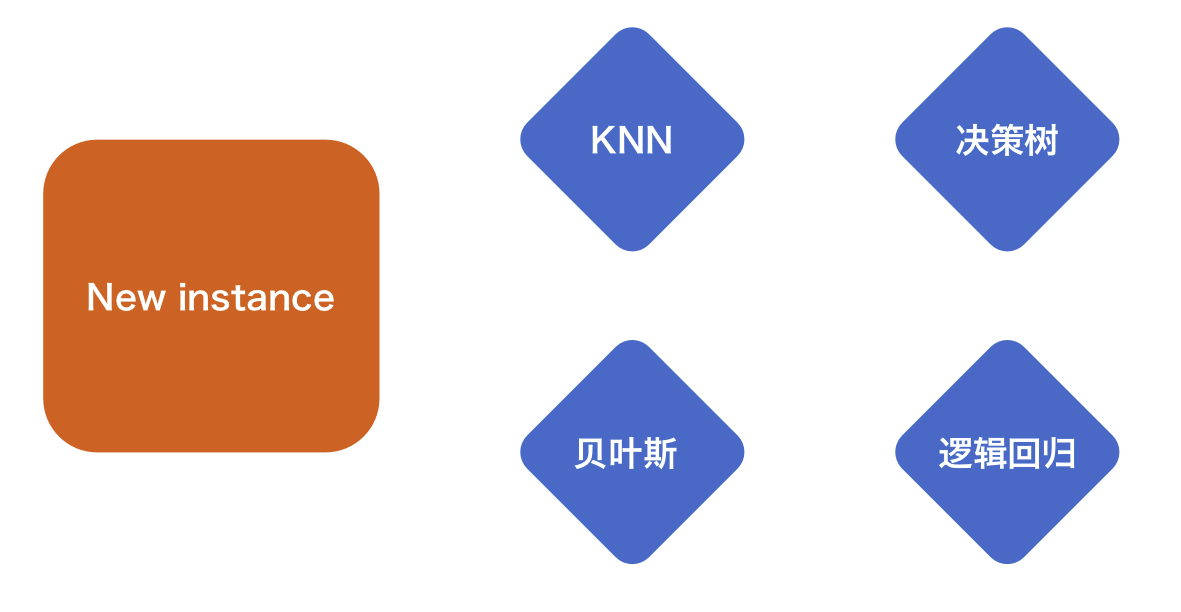Hivan Du

2023-11-05

2023-11-25